Given point R, not on line k, construct a line through R, perpendicular to k.

 1. Begin with point line k and point R, not on the line.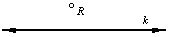2. Place the compass on point R. Using an arbitrary radius, draw arcs intersecting line k at two points. Label the intersection points X and Y.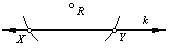3. Place the compass at point X. Adjust the compass radius so that it is more than (1/2)XY. Draw an arc as shown here.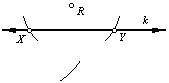4. Without changing the compass radius, place the compass on point Y. Draw an arc intersecting the previously drawn arc. Label the intersection point B.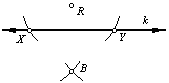5. Use the straightedge to draw line RB. Line RB is perpendicular to line k.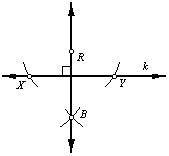Back to Geometry Construction Reference